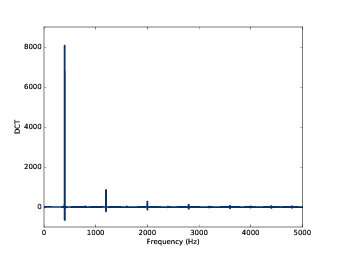# 第六章：离散余弦变换¶

DCT与频谱分解时用到的DFT很类似。学习了DCT之后可以更容易的理解DFT。

1. 先从合成信号开始：给定一组不同频率的余弦信号以及它们的幅值，构造一个合成的信号波形。
2. 使用Numpy来重写合成的函数，这样可以提升计算效率并且为下一步打好基础。
3. 分解信号：给定一个信号和每个频率分量，找到每个频率的幅值。我们先介绍一个简单直接的方法， 但是比较慢。
4. 最后，我们使用一些线性代数的知识来改善计算效率。如果你有线性代数的基础是最好的， 不过没有也没关系，我会把使用到的知识都简单介绍一下。

## 6.1 合成¶

def synthesize1(amps, fs, ts):
components = [thinkdsp.CosSignal(freq, amp)
for amp, freq in zip(amps, fs)]
signal = thinkdsp.SumSignal(*components)

ys = signal.evaluate(ts)
return ys


components 是一组由给定幅值和频率构造的余弦信号， SumSignal 计算出来这些信号的和。

amps = np.array([0.6, 0.25, 0.1, 0.05])
fs = [100, 200, 300, 400]
framerate = 11025

ts = np.linspace(0, 1, framerate)
ys = synthesize1(amps, fs, ts)
wave = thinkdsp.Wave(ys, framerate)


## 6.2 使用Numpy数组的合成¶

def synthesize2(amps, fs, ts):
args = np.outer(ts, fs)
M = np.cos(PI2 * args)
ys = np.dot(M, amps)
return ys


1. np.outer 计算了 tsfs外积（outer product） 。 外积的结果是一个n行m列的矩阵（n为ts的长度，m为fs的长度），其中的每个元素 都是对应位置的时间和频率的乘积 ft
2. args 乘以 $$2\pi$$ 并应用 np.cos 计算出了 $$\cos (2\pi ft)$$ 。也就是说 M 中的每一列都是一个频率分量 在 ts 时间序列下的值。
3. np.dot 计算出了 Mamps 的点积，也就是将 amps 的每个值与 M 中每行对应的值相乘并求和。这样就求出了每个时间的合成信号的值。$e = 0.6a + 0.25b + 0.1c + 0.05d$

ys1 = synthesize1(amps, fs, ts)
ys2 = synthesize2(amps, fs, ts)
max(abs(ys1 - ys2))


$\begin{split}M = \cos (2\pi t \otimes f)\\ y = Ma\end{split}$

## 6.3 分解¶

def analyze1(ys, fs, ts):
args = np.outer(ts, fs)
M = np.cos(PI2 * args)
amps = np.linalg.solve(M, ys)
return amps


n = len(fs)
amps2 = analyze1(ys[:n], fs, ts[:n])


[ 0.6   0.25  0.1   0.05 ]


## 6.4 正交矩阵¶

${M^{ - 1}}y = {M^{ - 1}}Ma$

${M^{ - 1}}y = Ia$

${M^{ - 1}}y = a$

def test1():
amps = np.array([0.6, 0.25, 0.1, 0.05])
N = 4.0
time_unit = 0.001
ts = np.arange(N) / N * time_unit
max_freq = N / time_unit / 2
fs = np.arange(N) / N * max_freq
ys = synthesize2(amps, fs, ts)


amps 是与之前一样的幅值向量。我们有4个频率成分，因此需要4个采样点就可以了，以保证 M 为方阵。

ts 是从0到1个单位时间的采样时间点序列，这里我随便选了一个0.001s作为单位时间，实际上无论选择什么 样的单位时间，计算结果都是一样的。

[[ 1.     1.     1.     1.   ]
[ 1.     0.707  0.    -0.707]
[ 1.     0.    -1.    -0.   ]
[ 1.    -0.707 -0.     0.707]]


[[ 4.  1. -0.  1.]
[ 1.  2.  1. -0.]
[-0.  1.  2.  1.]
[ 1. -0.  1.  2.]]


## 6.5 DCT-IV¶

def test2():
amps = np.array([0.6, 0.25, 0.1, 0.05])
N = 4.0
ts = (0.5 + np.arange(N)) / N
fs = (0.5 + np.arange(N)) / 2
ys = synthesize2(amps, fs, ts)


[[ 0.981  0.831  0.556  0.195]
[ 0.831 -0.195 -0.981 -0.556]
[ 0.556 -0.981  0.195  0.831]
[ 0.195 -0.556  0.831 -0.981]]


$${M^T}M$$ 等于:

[[ 2.  0.  0.  0.]
[ 0.  2. -0.  0.]
[ 0. -0.  2. -0.]
[ 0.  0. -0.  2.]]


def analyze2(ys, fs, ts):
args = np.outer(ts, fs)
M = np.cos(PI2 * args)
amps = np.dot(M, ys) / 2
return amps


def dct_iv(ys):
N = len(ys)
ts = (0.5 + np.arange(N)) / N
fs = (0.5 + np.arange(N)) / 2
args = np.outer(ts, fs)
M = np.cos(PI2 * args)
amps = np.dot(M, ys) / 2
return amps


ys 是波形数据，我们不用给定 tsfsdct_iv 会通过 ys 的长度来自行计算 tsfs

amps = np.array([0.6, 0.25, 0.1, 0.05])
N = 4.0
ts = (0.5 + np.arange(N)) / N
fs = (0.5 + np.arange(N)) / 2
ys = synthesize2(amps, fs, ts)
amps2 = dct_iv(ys)
max(abs(amps - amps2))


## 6.6 逆DCT¶

def inverse_dct_iv(amps):
return dct_iv(amps) * 2


inverse_dct_iv 其实就是信号的合成：它把输入幅值向量并输出合成信号 ys 。 我们可以像下面这样测试 inverse_dct_iv

amps = [0.6, 0.25, 0.1, 0.05]
ys = inverse_dct_iv(amps)
amps2 = dct_iv(ys)
max(abs(amps - amps2))


## 6.7 DCT类¶

thinkdsp 中提供了一个 Dct 类对DCT进行封装（类似于 Spectrum 类对FFT进行的封装）。 我们可以通过波形的 make_dct 来生成Dct对象:

signal = thinkdsp.TriangleSignal(freq=400)
wave = signal.make_wave(duration=1.0, framerate=10000)
dct = wave.make_dct()
dct.plot()make_dct 内部用的是DCT-II方法来计算DCT，在 scipy.fftpack 中提供这个方法:

import scipy.fftpack

# class Wave:
def make_dct(self):
N = len(self.ys)
hs = scipy.fftpack.dct(self.ys, type=2)
fs = (0.5 + np.arange(N)) / 2
return Dct(hs, fs, self.framerate)


dct 的结果保存在 hs 中，对应的频率值为 fs （见 6.5 DCT-IV ）。 然后使用它们和采样率来生成一个 Dct 对象。

Dct 类也提供了 make_wave 方法来计算逆DCT，我们来测试一下:

wave2 = dct.make_wave()
max(abs(wave.ys-wave2.ys))


wavewave2ys 差别也大概为1e-16（同样是浮点数误差）。

make_wave 使用了 scipy.fftpack.idct

# class Dct
def make_wave(self):
n = len(self.hs)
ys = scipy.fftpack.idct(self.hs, type=2) / 2 / n
return Wave(ys, framerate=self.framerate)


## 6.8 练习¶

1. 把长段的数据分段。
2. 计算每段的DCT。
3. 把幅值很小的频率成分去掉，保存剩下的频率和幅值。
4. 解压的时候，将频率和幅值进行逆DCT运算。